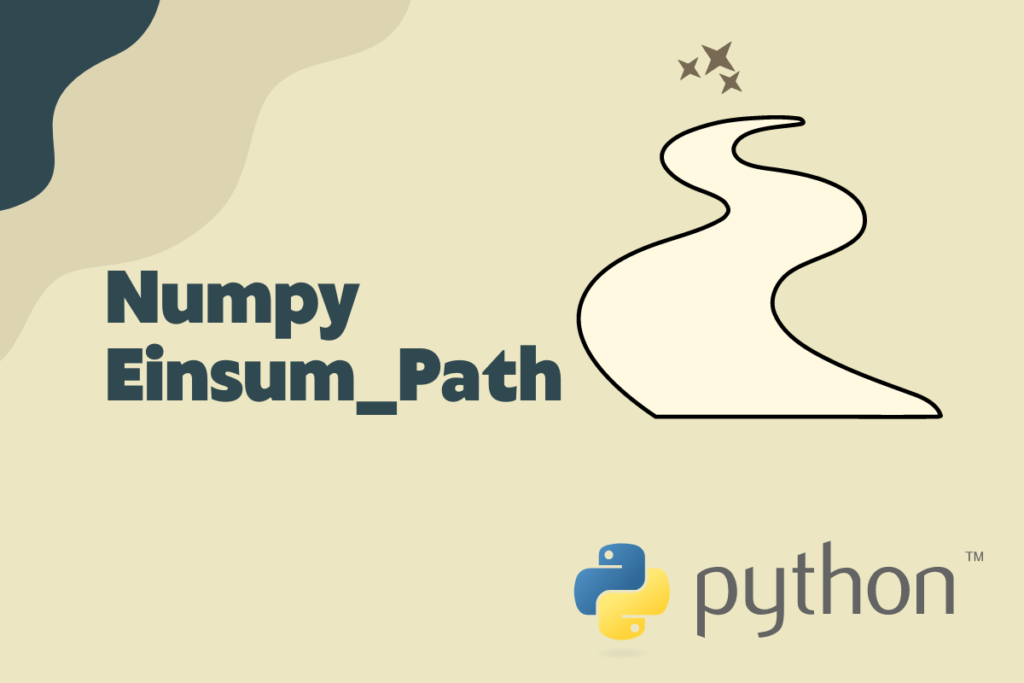# Mastering NumPy’s Powerful einsum_path( ) FunctionEinsum is an almighty function from the numpy library which is the most efficient manipulator of n-dimensional arrays. It can perform umpteen functions such as adding, multiplying or rearranging the input arrays in a jiffy, resulting in multiple x’s faster computation. Some implications of einsum might be interesting enough that they have no relation whatsoever with Einstein from the ein or summing from the sum.

One such implication would be the einsum_path( ) function which creates intermediate arrays for evaluating the lowest cost contraction order for an einsum expression. Rather than being bounded by performing contraction over similar dimensions, this function is capable of contracting any given dimension with a different dimension. The best part is that one can leverage this function to carry out contraction for any number of dimensions leaving aside those which one desires to be untouched.

## Syntax of the einsum_path( ) function

What else can be used to understand a function better, than its syntax? But the syntax of interest here might be a bit handful to comprehend. So, let us have a look at it.

```numpy.einsum_path(subscripts, *operands, optimize = ‘greedy’)
```

where

• subcripts – used to specify the subscripts that ought to be summed
• *operands – used to list the arrays that are to be used for the operation
• optimize – set to ‘greedy’ by default, it is used to specify the method to deduce the desired path type. The other path type that is available to choose is ‘optimal’

It is to be noted that the ‘greedy’ algorithm analyses each step and selects the most suitable contraction pair. The ‘optimal’ algorithm on the other hand tries out all the combinations thereby identifying every possible path to contract the inputs and selecting the order which is of the least cost. The einsum_path( ) function eventually returns the path in the form of a list.

## Putting einsum_path( ) into use

One shall get started by importing the numpy library to make use of the einsum_path( ) function.

```import numpy as np
```

Now let us declare the input arrays for which the path of contraction is to be determined.

```ar1 = np.array([[10, -9], [5, -7]])
ar2 = np.array([[0, 4, 6], [7, 5, -3]])
ar3 = np.array([[-1, 4], [6, 8], [-2, 4]])
```

It is time that we deploy the einsum_path( ) function for these arrays using the code below.

```pt = np.einsum_path('ij, jk, ki -> ii', ar1, ar2, ar3, optimize = 'greedy')
```

Ideally, the inputs ar2 and ar3 will be contracted first when the above code is executed and then the corresponding results shall be fed to deduce the final contraction.

```print(pt)
print(pt)
```

But what if one wants to try out their hand with the other algorithm for optimising the contraction path? Let us do that by setting the optimize option to ‘optimal’ as shown in the code below.

```pt = np.einsum_path('ij, jk, ki -> ii', ar1, ar2, ar3, optimize = 'optimal')
print(pt)
print(pt)
```